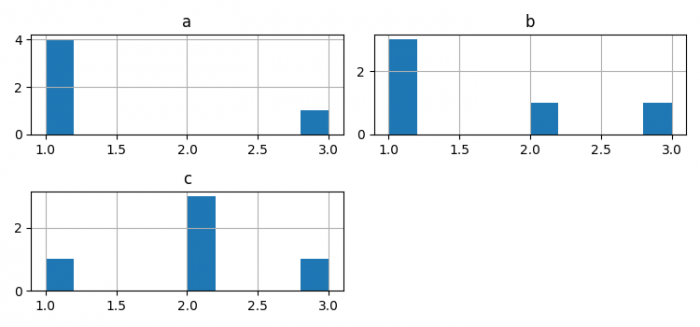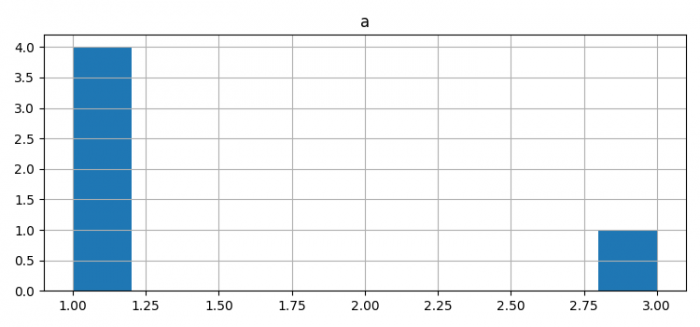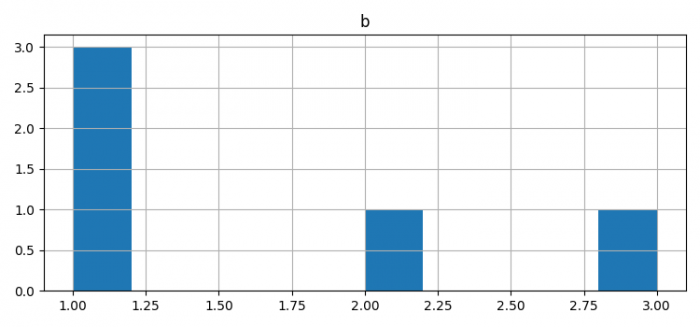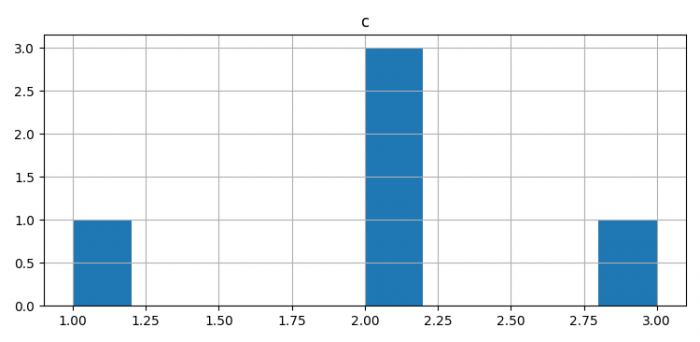# How to change the order of plots in Pandas hist command?

To change order of plots in Pandas hist commad, we can take the following steps −

• Make a data frame using Pandas.

• Plot a histogram with the data frame.

• Plot the data frame in different order.

• To display the figure, use show() method.

## Example

from matplotlib import pyplot as plt
import pandas as pd
plt.rcParams["figure.figsize"] = [7.50, 3.50]
plt.rcParams["figure.autolayout"] = True
df = pd.DataFrame({'a': [1, 1, 1, 1, 3],
'b': [1, 1, 2, 1, 3],
'c': [2, 2, 2, 1, 3],
})

df.hist()
df[['c']].hist()
df[['a']].hist()
df[['b']].hist()
plt.show()

## Output